# Application and Control of a Doubly Fed Induction Machine Integrated in Wind Energy System

Application and Control of a Doubly Fed Induction Machine Integrated in Wind Energy System
Department of Electrical Engineering, Faculty of Engineering Sciences, BP 89, Sidi Bel Abbes, Algeria

Corresponding Author Email:
fatimamoulay66@yahoo.fr
Page:
257-265
|
DOI:
https://doi.org/10.18280/i2m.180305
10 March 2019
|
Accepted:
15 May 2019
|
Published:
30 August 2019
| Citation

OPEN ACCESS

Abstract:

The global wind energy capacity has increased rapidly in this last decade and became the fastest developing renewable energy technology. But unbalances in wind energy are highly impacting the energy conversion and this problem can be resolved by a doubly Fed Induction Generator (DFIG) which is one of the most commonly deployed large grid-attached wind turbine systems. The objective of this paper is to study this system, as the first the mathematical model is developed in the coordinates of Park d-q, then the power transfer between the stator and the network is realized by action on the rotor signals via a bidirectional converter. Independent control of active-reactive power is provided by conventional controllers (PIs). Then, we realized the implementation of the control by an orientation of the rotor flux (FOC) which presents an attractive solution to have an operation with the best performances of the Machine, in the applications with variable speeds. The analysis of the simulation results under the Matlab / Simulink environment of this control approach clearly shows that the system perfectly follows the reference values and, consequently, provides a good static and dynamic performance of the machine under study.

Keywords:

doubly fed asynchronous machine, flux orientation command (FOC), wind turbine, doubly-fed induction generator, wind energy conversion system

1. Introduction

When it comes to electrical power, the world is seeking to significantly reduce its dependence on fossil fuels, characterized by both high greenhouse gas emissions and unstable prices. Operators in the electricity sector are striving to diversify their energy, especially through clean, renewable energy from geothermal, biomass, solar or wind sources .

One of the current areas of research is the generation of electrical energy using a doubly fed asynchronous machine, using the wind energy as a means of driving. Integrated in a wind system, the machine allows operating over a wide range of wind speeds, and to draw as much power as possible for each wind speed. Its stator circuit is connected directly to the electrical network.

A second circuit placed on the rotor is also connected to the network, but via power converters . And the transited rotor power is low, the cost of the converters is reduced compared to a variable-speed wind turbine that is fed to the stator by power converters.

This is the main reason why this machine is found for high power production. A second reason is the ability to adjust the voltage at the connection point of this machine.

In this paper a mathematical modeling of wind turbine has been simulated using MATLAB/SIMULINK and the results are analyzed for fixed and variable wind speed.

This paper presents a method of controlling a Doubly Fed Asynchronous Machine, it is organized as follows: an introduction in the first followed by a mathematical model of the set in the second, then the vector control and the results of the simulations under the Matlab environment / Simulink in the third and at last a conclusion.

2. Description and Modelling of the System

The overall system is illustrated in Figure 1 below, it consists of two converters (rectifier and inverter), one is connected to the stator and the other is connected to the rotor of the machine studied .

In order to control the electrical system, it is necessary to study and analyse its mathematical model taking into consideration certain hypotheses in order to obtain a simplified model .

## 1.jpgFigure 1. Wind energy conversion system based on a doubly fed asynchronous machine

2.1 Wind turbine modelling

2.1.1 Model of the wind

Wind turbine modelling requires the modelling of the wind, the aerodynamic behaviour of the blades, the electric generator, the power converter and the control system . Wind is the input variable of the wind system and wind speed is usually represented by a scalar function that changes over time, V = f (t).

The average wind speed is given by the following expression or Eq. (1) :

\begin{align} & {{V}_{moy}}=8+0.2\sin \left( 0.1047t \right)+2\sin \left( 0.2665t \right) \\ & +sin\left( 1.2930t \right)+0.2sin\left( 3.6645t \right) \\\end{align}       (1)

2.1.2 Aerodynamics

During the bibliography search, several models of the power coefficient were found, for example: 

Proposed the following model:

\begin{align} & {{C}_{p}}\left( \lambda ,\beta \right)=\left( 0.3-0.00167*\beta \right)\sin \left[ \frac{\pi \left( \lambda +0.1 \right)}{10-0.3\beta } \right] \\ & -0.00184.\left( \lambda -3 \right).\beta \\\end{align}   (2)

It is clear that the power coefficient in the equation (2) is a function of λ (The tip speed ratio), and β (The blade pitch angle in a pitch-controlled wind turbine).

## 2.pngFigure 2. Evolution of the wind turbine's power factor for different angles of adjustment β(°)

In Figure 2, several curves are distinguished but we are interested in the one with the highest peak. This curve is characterized by the optimal point $\left( \lambda _ { o p t } = 4.9 , C _ { p . \max } = 0.3 \beta = 0 \right)$ which corresponds to the maximum of the power coefficient (C p-max) and therefore the maximum of the mechanical power recovered.

The power produced by a wind crossing a surface S depends on the cube of the wind speed $\mathrm { V }$ and the density of the air $\rho$ . This power is given by the Eq. $( 3 )$ :

$P _ { v } = \frac { 1 } { 2 } \cdot \rho . S . V ^ { 3 }$   (3)

The mechanical power available on the rotor $\mathrm { Pm }$ is generally expressed using the power coefficient and is given by Eq. $( 4 ) :$

$P _ { m } = \frac { 1 } { 2 } \cdot \rho . S \cdot C _ { P } ( \lambda , \beta ) . V ^ { 3 }$   (4)

Taking into account the ratio of the speed multiplier, the mechanical power $P m$ available on the shaft of the electric generator is given by the Eq. $( 5 )$ :

$P m = \frac { 1 } { 2 } C _ { P } \left( \frac { \Omega _ { 2 } R } { K V _ { v } } \right) p . \pi . R ^ { 2 } V ^ { 3 }$   (5)

where,

Cp: The aerodynamic coefficient of power.

R: Radius of the wind turbine 35.25m

ρ: Density of the air 1.225 $K g . m ^ { - 1 }$

This equation allows to establish a set of characteristics giving the power available according to the speed of rotation of the generator for different wind speeds.

2.1.3 Gear box model

The gear box adapts the speed (slow) Ω1 from turbine to speed (fast) Ω2 of the generator. This gear box is modelled mathematically by the following expressions:

${{\text{ }\!\!\Omega\!\!\text{ }}_{2}}=K.{{\text{ }\!\!\Omega\!\!\text{ }}_{1}}$     (6)

where,

Ω2: rotational speed after the gear box

Ω1: Speed of rotation before the gear box

The mass of the wind turbine is transferred to the turbine shaft in the form of an inertia Jturbin and includes the mass of the blades and the rotor mass of the turbine. The proposed mechanical model considers total inertia Jtotal consisting of the inertia of the turbine reported on the rotor of the generator and the inertia of the generator.

$J={{J}_{total}}=\frac{{{J}_{turbin}}}{{{K}^{2}}}+{{J}_{g}}$         (7)

2.2 Modelling of the machine

The dynamic model of the Doubly Fed Asynchronous Machine, in d-q frame can be represented by the following Eq. (8) and Eq. (9): 

2.2.1 Electrical equations

\left\{ \begin{align} & {{V}_{sd}}={{R}_{s}}.{{I}_{sd}}+\frac{d{{\varphi }_{sd}}}{dt}-{{\varphi }_{sq}}.{{\omega }_{0}} \\ & {{V}_{sq}}={{R}_{s}}.{{I}_{sq}}+\frac{d{{\varphi }_{sq}}}{dt}-{{\varphi }_{sd}}.{{\omega }_{0}} \\ & {{V}_{rd}}={{R}_{r}}.{{I}_{rd}}+\frac{d{{\varphi }_{rd}}}{dt}-{{\varphi }_{rq}}.\left( {{\omega }_{0}}-{{\omega }_{r}} \right) \\ & {{V}_{rq}}={{R}_{r}}.{{I}_{rq}}+\frac{d{{\varphi }_{rq}}}{dt}-{{\varphi }_{rd}}.({{\omega }_{0}}-{{\omega }_{r}}) \\ & {{\omega }_{0}}=\frac{d{{\theta }_{0}}}{dt} \\\end{align} \right.            (8)

2.2.2 Magnetic equations

\left\{ \begin{align} & {{\varphi }_{ds}}={{L}_{s}}{{I}_{ds}}+M{{I}_{dr}} \\ & {{\varphi }_{qs}}={{L}_{s}}{{I}_{qs}}+M{{I}_{qr}} \\ & {{\varphi }_{dr}}={{L}_{r}}{{I}_{dr}}+M{{I}_{ds}} \\ & {{\varphi }_{qr}}={{L}_{r}}{{I}_{qr}}+M{{I}_{qs}} \\\end{align} \right.        (9)

2.2.3 Mechanical equation

The electromagnetic torque of a three-phase asynchronous machine modelled in the ‘Park coordinate system’ is given by the following Eq. (10):

${{C}_{em}}=P.{{M}_{sr}}\left( {{i}_{qs}}.{{i}_{dr}}-{{i}_{ds}}.{{i}_{qr}} \right)$            (10)

The reactive and active powers at the stator can be expressed by the Eq. (11):

\left\{ \begin{align} & {{P}_{s}}={{v}_{ds}}{{i}_{ds}}+{{v}_{qs}}{{i}_{qs}} \\ & {{Q}_{s}}={{v}_{ds}}{{i}_{qs}}-{{v}_{qs}}{{i}_{ds}} \\\end{align} \right.      (11)

The rotor currents that provide independent control of the active power Ps and the reactive power Qs of the DFIG must be defined in the stator flux-oriented reference frame.

2.3 Modelling of electricity conversion components

2.3.1 Structure of the Back-to-Back converter

The power structure, called Back-to-Back is now widely used to adapt the frequency of the rotor voltages to that of the stator circuit and therefore the network. Its use is justified by the fact that it allows operating on four quadrants, which means that the direction of the energy transition can be reversed at any time. Consequently, the two voltage converters used can operate in rectifier mode or in inverter mode, whether they are connected on the rotor side or on the grid side, and the DC bus is therefore reversible .

Each of the two converters has three switching arms, six bidirectional electronic switches, controllable at the opening and closing, and an IGBT type transistor connected head to tail with diodes. The control strategy of the converters is based on the Pulse Width Modulation (PWM) technique, which makes it possible to limit the harmonics by reducing the latter’s content at low frequency .

## 3.pngFigure 3. Back-to-Back structure of power converters

2.3.2 Operation principle of a PWM rectifier

Interposed between the rotor of the Machine and the network are two bi-level, PWM type converters that are bidirectional in power. The equivalent model of the converters represented in the Park mark, the DC bus, the link to the network containing the second PWM converter, the intermediate filter and the network connection node are adopted .

In our case, the converter connected to the rotor will operate as a rectifier, and the one connected to the network will operate as an inverter. This allows us to recover the electrical power available at the rotor winding through the sliding contacts and reinject it into the network. . This type of converter can operate as a rectifier or inverter. When the current $i _ { r e s }$ is positive (rectifier operation), the capacitor C is discharged, and the error signal requests the control block for more energy from the network, the control block takes the power supply by producing signals suitable for priming the transistors. In this way, the current flows from the AC side to the DC side, thus, the capacitor voltage is recovered. Conversely, when $i _ { r e s }$ becomes negative (Inverter Operation), the capacitor C is overloaded, and the error signal induces the controller to discharge the capacitor and return the energy to the network .

The control signals of the rotor-side converter come from the application of the generator’s vector control whose network-side convertor is responsible for adjusting the voltage of the DC bus to always supply power to the first regardless of the direction of transit power .

This voltage setting of the DC bus sets the reference active power to be exchanged with the network via the intermediate R, L filter. Unit-side factor is used for this, and a reactive reference equal to zero is set . It is these two instructions that will serve us to impose the reference currents transited to the network .

2.4 Modeling of the continuous bus

The DC bus must be maintained at a constant voltage, it comprises a capacitor which acts as a storage during the exchange of energy and makes it possible to limit the undulation of the DC voltage .

## 4.pngFigure 4. Block diagram of the continuous bus

The time evolution of the DC bus voltage is obtained from the integration of the capacitive current:

$\frac{d{{v}_{c}}}{dt}=\frac{1}{C}.{{i}_{c}}$          (12)

Therefore, the DC bus voltage can be expressed as follows:

${{v}_{dc}}=\int ~\frac{1}{C}.{{i}_{c}}.dt$       (13)

The current of the capacitor is coming from a node where the currents $i _ { m a c }$ and $i _ { r e s }$  modulated by the converters circulate:

$~{{i}_{c}}={{i}_{mac}}-{{i}_{res}}$         (14)

The currents transited between the converter and the network are imposed by the coils constituting the low pass filter . The voltage across the filter is given by:

$\left[ \begin{matrix} {{v}_{m1}} \\ {{v}_{m2}} \\ {{v}_{m3}} \\\end{matrix} \right]={{R}_{f}}.\left[ \begin{matrix} {{i}_{t1}} \\ {{i}_{t2}} \\ {{i}_{t3}} \\\end{matrix} \right]+{{L}_{f}}.\frac{d}{dt}\left[ \begin{matrix} {{i}_{t1}} \\ {{i}_{t2}} \\ {{i}_{t3}} \\\end{matrix} \right]+\left[ \begin{matrix} {{v}_{s1}} \\ {{v}_{s2}} \\ {{v}_{s3}} \\\end{matrix} \right]$     (15)

By applying Park's transformation to the synchronous reference, the preceding equation becomes:

$\left\{ \begin{matrix} {{v}_{md}}={{R}_{f}}.{{i}_{td}}+{{L}_{f}}.\frac{d{{i}_{td}}}{dt}-{{\omega }_{s}}.{{L}_{f}}.{{i}_{tq}}+{{v}_{sd}} \\ {{v}_{mq}}={{R}_{f}}.{{i}_{td}}+{{L}_{f}}.\frac{d{{i}_{td}}}{dt}-{{\omega }_{s}}.{{L}_{f}}.{{i}_{td}}+{{v}_{sq}} \\\end{matrix} \right.~$        (16)

3. Simulation and Results

In this part of the work, we present the results of the simulation using the MATLAB / SIMULINK tool for the control of the asynchronous machine with dual power supply, Integrated in the wind energy conversation chain.

In this simulation we use the MPPT control and FOC command strategies .

## 5a.png(a)

## 5b.jpg(b)

Figure 5. Wind model diagram on MATLAB/ simulink

## 6.png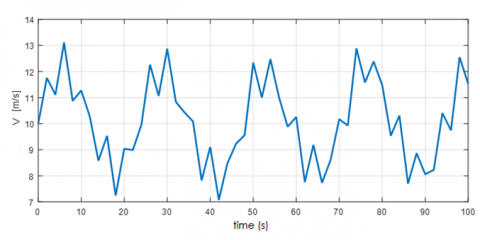Figure 6. Wind profile curve

To improve system efficiency, turbine speed is adjusted as a function of wind speed to maximize output power.

For these simulations, we use the average wind speed of 11 m/s. This curve simulates the small variations of the wind speed around an average value to get as close as possible to reality .

3.2 simulations of the turbine

The turbine is driven by a Doubly Fed Asynchronous Machine, the block diagram corresponding to this model of the turbine is shown in Figure 7.

The Figure 8 shows the torque variation curve of the turbine after the gear box Cm in function of time. It is clear that this torque decreases until it stabilizes at t=2.15s.

Figure 9 shows a curve of the aerodynamic torque variations of the turbine in function of time. These variations decrease until the instant of stabilization 2.15 s.

Figure 10 shows a curve of the turbine power factor variations in function of time which increase up to the instant of stabilization t=2.15s at the value CMax=0.45 at a wedging angle β=0 °.

## 7.png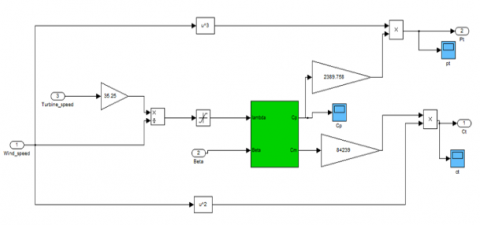Figure 7. Wind turbine implementation diagram on MATLAB / simulink

## 8.pngFigure 8. Torque curve of the turbine

## 9.pngFigure 9. Turbo dynamic torque curve

## 10.png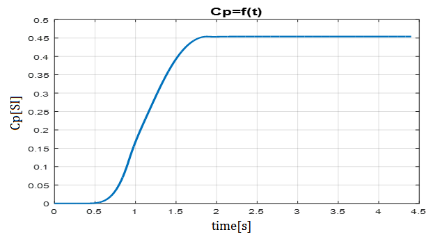Figure 10. Curve of the power coefficient of the turbine

## 11.pngFigure 11. Curve of the specific speed λ

Figure 11, represents a curve of the specific velocity variations λ of the turbine in function of time. These variations increase until the moment of simulation 2.15s stabilizes with the value  λcp max=7.1 at a wedging angle β=0 °.

The value of λ corresponding to maximum of mechanical power available is called λopt (optimal). The rotor torque istained from the power received and the speed of rotation of the turbine.

3.3 Simulation of the global chain

The purpose of the wind system is to convert the kinetic energy of the wind into available mechanical energy on a transmission shaft, and then into electrical energy through a generator.

The overall diagram of a wind energy conversion chain connected to the electricity grid is described by the figure 12

In order to simulate the control system, MATLAB / SIMULINK software was used. The machine parameters are given in the appendix.

The results shown in the figures below are those obtained for the model of a twin-power asynchronous machine with a power of 20 MW.

Figure 13 represents the variation curve. The voltage VDC reaches the reference value of 1150V in 2.15 seconds without exceeding it. The static error is then zero once the steady state reached.

## 12.pngFigure 12. Block diagram of the simulation of the doubly fed asynchronous machine with control

## 13.png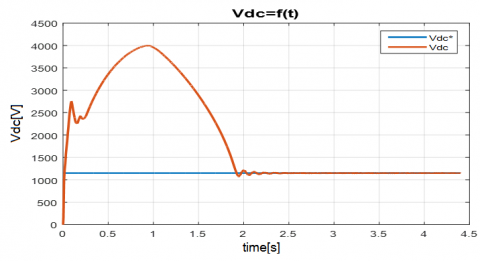Figure 13. Curve of the converters voltage

## 14.png## 14b.png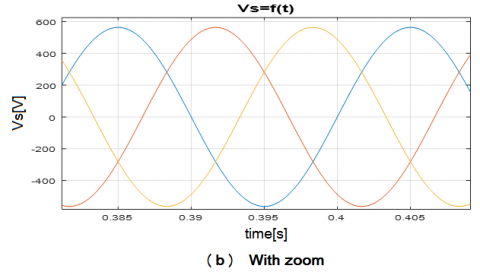Figure 14. Curve of the stator voltage

## 15.png## 15b.pngFigure 15. Curve of the stator current

Figure 14 shows a curve of the stator voltage variations in function of time. These variations are constant in time.

Figure 15, represents a curve of the current variations in function of time. These variations are constant after t=2.15s of the simulation.

Figure 16, shows a curve of the rotor-current variations in function of time. These variations are constant after t=2.15s.

Figure 17, shows a curve of the rotor current variations on the park axis d and the rotor current on the reference axis d in function of time. These variations of rotor-current Idr  are increasing up t=2.15s then it stabilises.

## 16.png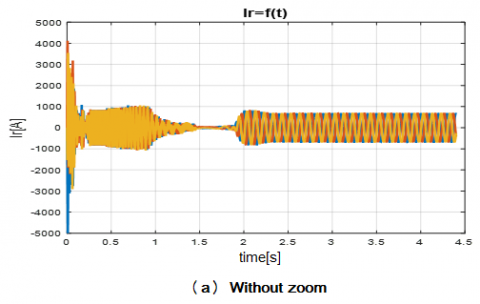## 16b.pngFigure 16. Rotor current curve

## 17.png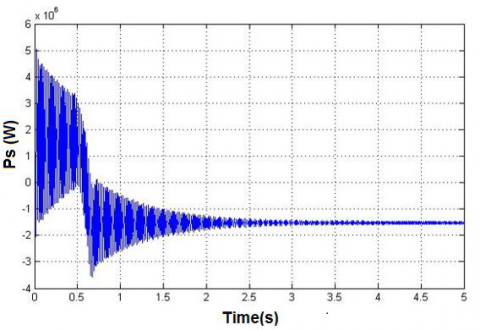Figure 17. Curve of the rotor current on the axis

## 18.pngFigure 18. Curve of the rotor current on the axis q

Figure 18 shows a curve of the rotor current variations on the park q axis and rotor current on the park d reference axis in function of time. These variations start stabilising on the q axis around the value of the reference $I _ { q r } ^ { * }$.

## 19.pngFigure 19. Electromagnetic torque curve machine

## 20.pngFigure 20. Machine speed curve

Figure 19, shows a curve of the electromagnetic torque and the reference electromagnetic torque in function of time. These variations of Cem are decreased until t=2.15s around the value of Cem*.

Figure 20, represents a curve of the variations of speed and reference speed in time. The speed Ω is increasing until t=2.15s then it stabilizes around the reference speed Ω*.

4. Conclusion

According to the optimal power characteristic of the wind turbine, it shows that for each wind speed, there is an optimal rotation speed, corresponding to the maximum value of the power coefficient allowing the wind turbine to have the best possible efficiency. To be able to rotate at this optimum speed, at the given wind speed, the turbine must have a given resistant mechanical torque, which means an active power delivered by the Doubly Fed Asynchronous Machine or Generator (DFIG).

We also introduced the mathematical model of the doubly fed induction generator dedicated for the variable speed wind turbines; with aim to controlling the stator active and reactive power of the DFIG connected to the public network, we have applied the stator field oriented control strategy. Also, the simulation results were presented using the Back-to-Back converter. Finally, we concluded that the (FOC) control gives good results.

Again controlling the DFIG from the rotor side makes the control process more cost effective as the rotor converters have to deal with comparatively less power when connected at the rotor side than when connected at the stator side ,we can used another type of control like the sliding mode or nonlinear control to give better performance as perspective.

Nomenclature
 DFIG Doubly Fed Induction Generator Vsd,Vsq,Vrd,Vrq Stator and rotor voltage components in the d-q reference frame. ϕsd, ϕsq, ϕrd, ϕrq, Stator and rotor flux components in the d-q reference frame. Isd,Isq,Ird,Irq Stator and rotor current components in the d-q reference frame. ω0, ωr Stator frequency, rotor rotating speed. Rs, Rr Stator-Rotor resistances. Ls,Lr Stator and Rotor inductance respectively. M Mutual inductance. Ps,Qs Active reactive stator power respectively p Number of pole pairs. Cem Electromagnetic torque Pm Echanical power S Section of blade Cp The aerodynamic coefficient of power. R Radius of the wind turbine f Friction coefficient Ω1: Speed of rotation before the gear box Ω2 rotational speed after the gear box J Inertia moment Greek symbols ρ Density of the air λ The tip speed ratio β The blade pitch angle φ flux Ѳ0 Rotor angle
Appendix

System settings

Rated power: $P n = 4 k W$

Rated voltage: $v / U = 220 / 380 \mathrm { V } - 50 \mathrm { Hz }$

The nominal speed: $\Omega n = 1440 \mathrm { tr } / \mathrm { min }$ .

Number of pole pairs: $P = 2$

The parameters of the wind turbine used:

Number of blades: $N p = 3$

Diameter of a blade: $R T = 3 m$

Inertia: $J = 315 \mathrm { Kg } \cdot \mathrm { m } 2$

Number of blades: $N p = 3$

Stator resistance: $\mathrm { Rs } = 1.2 \Omega$

Rotor resistance: $R \mathrm { r } = 1.8 \Omega$

Stator inductance: $L s = 0.1554 H$

Rotor inductance: $L r = 0.1568 H$

Mutual inductance: $M = 0.15 H$

Mechanical constants:

Moment Inertia: $J = 0.2 \mathrm { Kg } . m ^ { 2 }$

Coefficient of friction: $f = 0.001 \mathrm { N.m. } \mathrm { S } / \mathrm { rad }$

References

 Lampola, P. (2000). Direct driven, low speed permanent magnet generator for wind power applications. Acta Politechnica Scandinaviica, Electrical Engineering Series, N°101, ESPOO; Published by the Finnish Academies of Technology.

 Tapia, G., Tapia, A., Ostolaza, J.X. (2007). Proportional-integral regulator-based approach to wind farm reactive power management for secondary voltage control. IEEE Transactions on Energy Conversion, 22(2): 488-498.

 Jirutitijaroen, P. (2010). Average power in the wind and energy estimate lectures notes on sustainable energy systems. National University of Singapore.

 Meroufel, A., Djeriri, Y., Massoum, A., Hammoumi, A. (2010). Commande vectorielle par les réseaux de neurones artificiels de l’énergie d’une MADA intégrée à un système éolien. Revue des Energies Renouvelables, 13(4): 669-682.

 Ahmed Shata, A. (2010). Wind energy as a potential generation source at Ras Benas. Egypt. Renewable and Sustainable Energy Reviews, 14(8): 2167-2173. https://doi.org/10.1016/j.rser.2010.03.006

 Serhoud, H., Benattous, D. (2011). Sensorless sliding power control of doubly fed induction wind generator based on MRAS observer. World Academy of Science, Engineering and Technology, 56: 920-925.

 Aksas, M., Gama, A. (2011). Assessment of wind and solar energy resources in Batna, Algeria. Energy Procedia, 6: 459-466. https://doi.org/10.1016/j.egypro.2011.05.053

 Aksas, M., Gama, A. (2011). Assessment of wind and solar energy resources in Batna, Algeria. Energy Procedia, 6: 459-466. https://doi.org/10.1016/j.egypro.2011.05.053

 Taoussi, M., Karim, M., Bossoufi, B., Lagrioui, A., El Mahfoud, M. (2013). The fuzzy control for rotor flux orientation of the double-fed asynchronous generator drive. International Journal of Computers & Technology, 13(8). https://doi.org/10.24297/ijct.v13i8.7069

 De Andrade, C.F., Falcão Maia Neto, H., Alexandre Costa Rocha, P., da Silva, M.E.V. (2014). An efficiency comparison of numerical methods for determining Weibull parameters for wind energy applications: A new approach applied to the northeast region of brazil. Energy Conversion and Management, 86: 801-808. https://doi.org/10.1016/j.enconman.2014.06.046

 Allam, M., Dehiba, B., Abid, M., Djeriri, Y., Adjoudj, R. (2014). Etude comparative entre la commande vectorielle directe et indirecte de la Machine Asynchrone à Double Alimentation (MADA) dédiée à une application éolienne. Journal of Advanced Research in Science and Technology, 1(2): 88-100.

 Djeridi, Y. (2015). Artificial neural network-based robust tracking control for doubly fed induction generator used in wind energy conversion systems. Journal of Advanced Research in Science and Technology, 2(1): 173-181.

 Bedouda, K., Ali-rachedi, M., Bahi, T., Lakel, R., Grid, A. (2015). Robust control of doubly fed inductiongenerator for wind turbine under sub-synchronous operation mode. Energy Procedia, 74: 886-899. https://doi.org/10.1016/j.egypro.2015.07.824

 Khatounian, F., Dehiba, B., Abid, M., Djeriri, Y., Adjoudj, R. (2016). Control of a doubly fed induction generator for aircraft application. Records of IEEE IECON 2003, Roanoke, USA, p. 20711. https://doi.org/10.1109/IECON.2003.1280676

 Amrane, F., Chaiba, A. (2016). A novel direct power control for grid-connected doubly fed induction generator based on hybrid artificial intelligent control with space vector modulation. Rev. Roum. Sci. Techn.-Electrotechn. Et Energ, 61(3): 263-268.

 Benkahla, M., Taleb, R., Boudjema, Z. (2016). Comparative study of robust control strategies for a dfigbased wind turbine. International Journal of Advanced Computer Science and Applications, 7(2): 455-462.https://doi.org/10.14569/IJACSA.2016.070261

 Boudjema, Z., Taleb, R., Djeriri, Y., Yahdou, A. (2017). A novel direct torque control using second order continuous sliding mode of a doubly fed induction generator for a wind energy conversion system. Turkish Journal of Electrical Engineering and Computer Science, 25: 965-97. https://doi.org/10.3906/elk-1510-89

 Amirinia, G., Mafi, S., Mazaheri, S. (2017). Offshore windresource assessment of Persian Gulf using uncertainty analysis and GIS. Renewable Energy, 113: 915-929. https://doi.org/10.1016/j.renene.2017.06.070

 Khan, K.S., Tariq, M. (2018). Wind resource assessment using sodar and meteorological mast a case study of Pakistan. Renewable and Sustainable Energy Reviews, 81: 2443-2449. https://doi.org/10.1016/j.rser.2017.06.050

 Abderrahim, A., Ghellai, N., Bouzid, Z., Menniand, Y. (2019). Wind energy resource assessment in south western of Algeria. Mathematical Modelling of Engineering Problems, 6(2): 157-162.https://doi.org/10.18280/mmep.060201

 Ahmed Shata, A. (2010). Wind energy as a potential generation source at Ras Benas, Egypt. Renewable and Sustainable Energy Reviews, 14(8): 2167-2173. https://doi.org/10.1016/j.rser.2010.03.006

 Zouggar, E.O., Chaouch, S., Ould Abdeslam, D., Abdelhamid, A.L. (2019). Sliding control with fuzzy type-2 controller of wind energy system based on doubly fed induction generator. Instrumentation Mesure Metrologie, 18(2): 137-146 https://doi.org/10.18280/i2m.180207

 Berkani, A., Negadi, K., Allaoui, T., Mezouar, A., Denai, M. (2019). Imposed switching frequency direct torque control of induction machine using five level flying capacitors inverter. European Journal of Electrical Engineering, 21(2): 241-284. https://doi.org/10.18280/ejee.210217